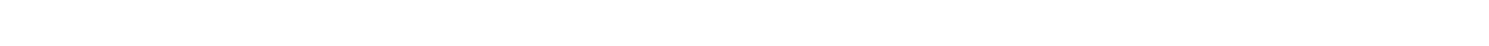# Calculate pH of Weak Acid

In this JC2 webinar we want to learn how to calculate the pH of a weak acid.

Let's take a look at this question.We know that this acid is weak since its acid dissociation constant Ka is given.

Note that strong acids are fully dissociated hence do not have Ka values.

We can write down the dissociation of the acid via the ICE table.ICE stands for Initial, Change and Equilibrium.

Since the degree of dissociation is not known, we can let it be x.

Hence we can determine the equilibrium concentrations of CH3COOH, CH3COO- and H+ in terms of x.

Next we can substitute these values into the Ka expression.Since Ka is known, we now have an equation in terms of x, and we can solve for it.

However the equation is quadratic so we have to do an approximation to simplify the calculation.

Note that for A Level Chemistry Syllabus, solving for quadratic equations is not required.

The approximation is pretty simple. Since the degree of dissociation is small, we assume the concentration of the acid at equilibrium is equal to its initial concentration.We can now rewrite the Ka expression into a non-quadratic equation.Now we can calculate x, which is the concentration of H+, and the pH.Most of the weak acids in Ionic Equilibria are monoprotic with small degree of dissocation.

Hence the ICE table and approximation mentioned here will be applicable most of the time.

This means we can just use the following formula to calculate H+ concentration directly.Topic: Ionic Equilibria, Physical Chemistry, A Level Chemistry, Singapore

Back to other previous Physical Chemistry Video Lessons.

Found this A Level Chemistry video useful?